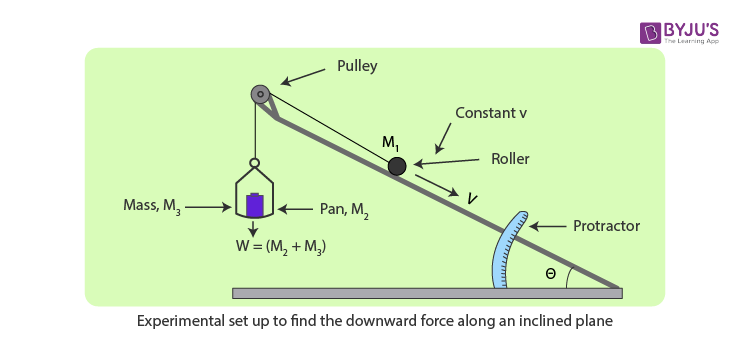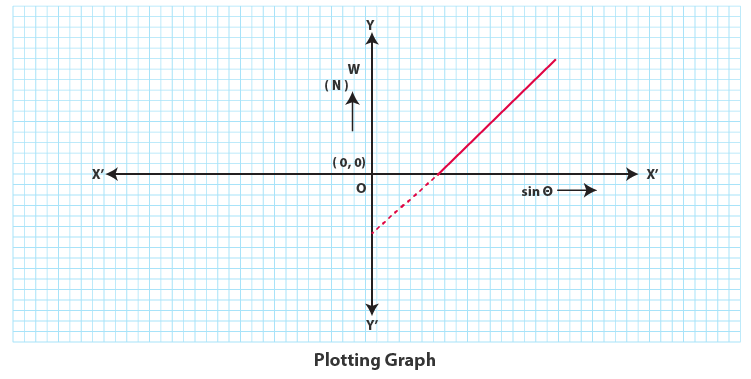# To find the downward force, along an inclined plane, acting on a roller due to the gravitational pull of the earth and study its relationship with the angle of inclination (θ) by plotting graph between force and sin θ

## Aim

To determine the downward force, along an inclined plane, acting on a roller due to gravity and to study its relationship with the angle of inclination by plotting graph between force and sin θ.

## Apparatus/ Materials Required

• Inclined Plane With Protracter And Pulley
• Weight box
• Roller
• Spirit Level
• Spring Balance

## Diagram## Principle

In the figure, we can see that a roller of mass M1 has been placed on an inclined plane making an angle θ with the horizontal. An upward force along the inclined plane is applied to the body by adjusting the suspended weights. The other end of the string is attached to the mass through a pulley fixed at the top of the plane. The force on the mass M1 when moving with a constant velocity v is given by the expression

W = M1g sin θ – fr

where fr is the force of friction due to rolling,

M1 is the mass of the roller,

W is the total tension in the string (W = Weight suspended).

## Procedure

1. Arrange the inclined plane, masses and roller as shown in the diagram. Make sure that the pulley is frictionless. Lubricate it using a machine if required.
2. Adjust the suspended W such that the roller stays at the top of the plane when at rest.
3. Slowly, start decreasing the mass in the pan until the roller starts moving down the plane with a constant velocity. Note down the value of W and the angle θ.
4. Repeat steps 2 and 3 for different values of θ and tabulates your observations.

## Observation

Mass of the roller = M1 g

Mass of the pan = M2 g

Acceleration due to gravity = …..N/m2

 S. No θ0 Sinθ Mass added to the pan M3 Force W = (M2 + M3)g N 1 2 3

## Plotting Graph

Plot the graph between sinθ and the force W. It should be a straight line.## Result

Therefore, within experimental error, downward force along an inclined plane is directly proportional to sin θ, where θ is the angle of inclination of the plane.

## Viva Voce

1. Explain the relationship between the downward force and the angle of inclination of the plane.Answer: The relation between the downward force and the angle of inclination is given by
mg sin θ. For a constant mass m, W∝Sinθ.
2. What is an inclined plane?Answer: An inclined plane is a wooden plane over which a glass sheet is placed to make the surface smooth. It makes an angle θ with the horizontal ground.
3. What is the normal reaction on the inclined plane?Answer: The normal reaction on the inclined plane is mgcosθ.
4. List a few applications of the inclined plane.Answer: Following are a few applications of the inclined plane:
• Used in the ramp of the house
• The principle of inclined plane used in the way we use the ladder
• A plank put at the back of the truck to load and unload heavy goods

Stay tuned with BYJU’S to get the latest notification on CBSE along with CBSE syllabus, sample papers, marking scheme and more.

Test Your Knowledge On To Find Downward Force Along Inclined Plane On A Roller Due To Gravitational Pull Of The Earth And Its Relationship With The Angle Of Inclination!